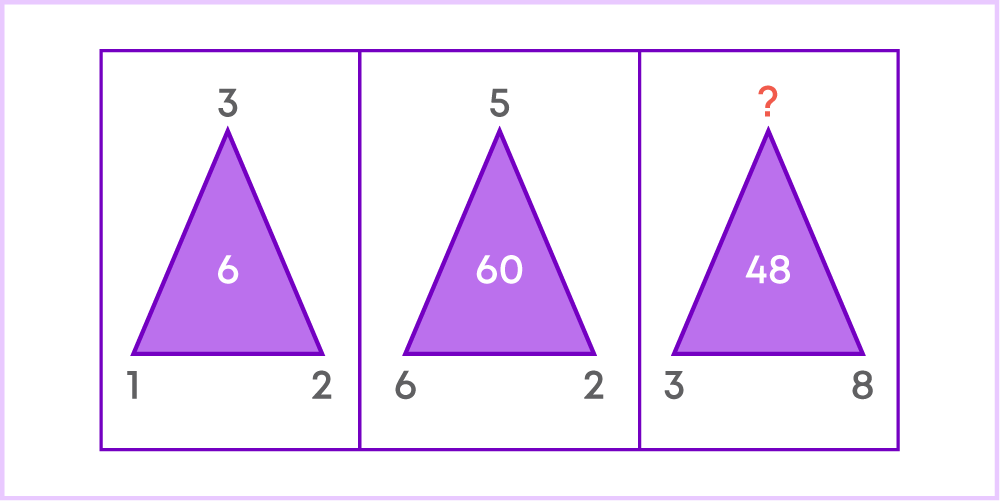## Introduction

Do you enjoy solving number puzzles? Exploring numbers in detail helps us to understand their amazing properties.

Did you know there are two numbers who are best friends? Watch the video to find out the relationship among numbers.

The video helped us to know that numbers have interesting relationships. In this chapter, we will explore more about them.

#### Concepts

The chapter ‘Playing With Numbers’ covers the following concepts:

#### Factors and Multiples

A factor of a number is an exact divisor of that number. Eg: Factors of 6 are 1, 2, 3, and 6.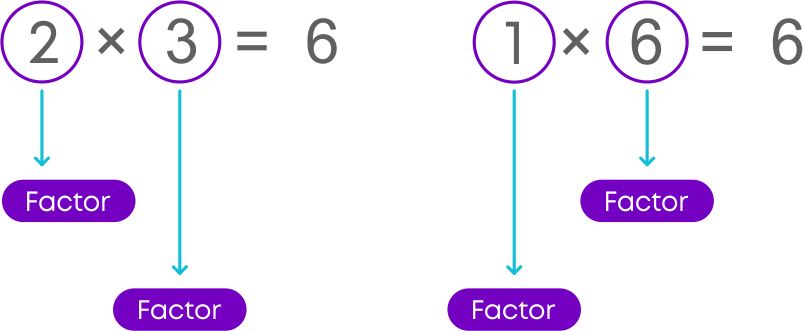Some properties of factors of a number are:

• 1 is a factor of every number
• Every number is a factor of itself
• Every factor is less than or equal to the given number
• The number of factors of a given number is finite

Did you know? A number for which sum of all its factors is equal to twice the number is called a perfect number. 6 is the first perfect number.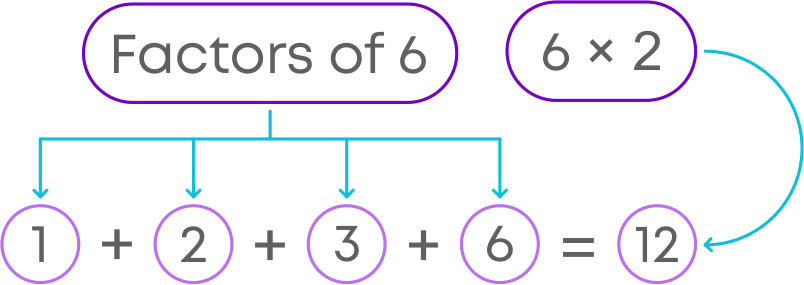The first 5 perfect numbers are:

• 6
• 28
• 496
• 8,128
• 3,35,50,336

Now, a multiple of a number is the product of that number and another number. The numbers that appear in the times/multiplication table of a number are its multiples. For example, the multiples of 3 are 3, 6, 9, 12, 15,… etc.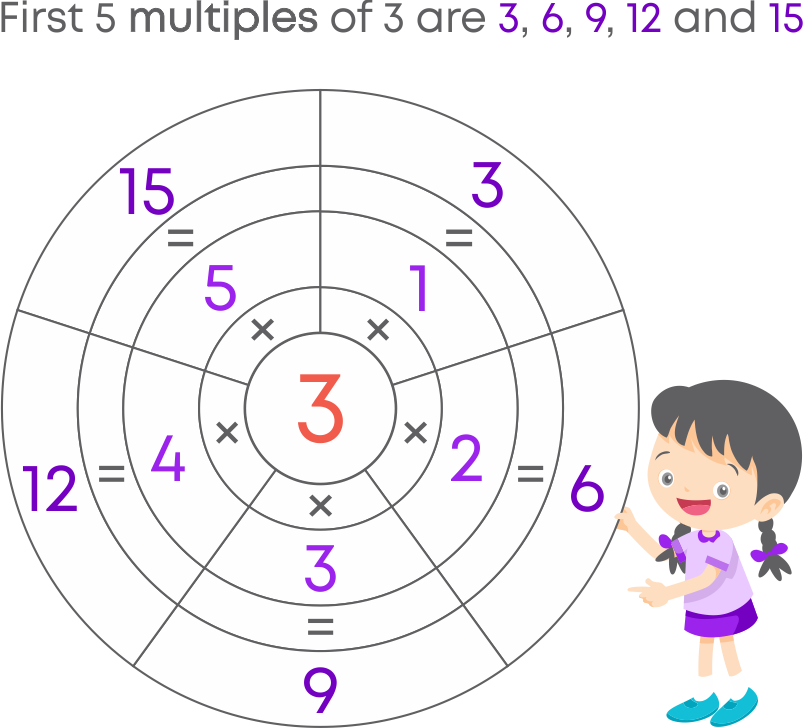Remember, any number is a multiple of each of its factors, and any number is a multiple of itself since any number can be a product of 1 and itself.

Let us conclude the properties of multiples of a number:

• Every number is a multiple of itself
• Every multiple is greater than or equal to the given number
• The number of multiples of a given number is infinite

Do you know how factors and multiples are related? Any number is a multiple of each of its factors. For example, 3 × 5 = 15.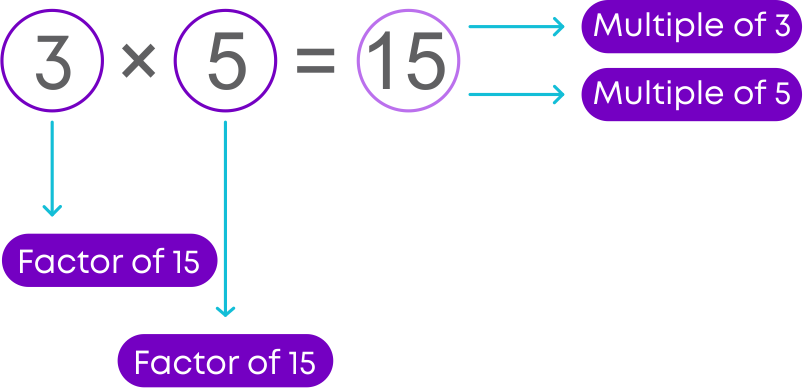• 3 and 5 are the factors of 15
• 15 is a multiple of both 3 and 5

#### Special Properties of Numbers

Let us learn some interesting terms about numbers:

• The numbers whose only factors are 1 and the number itself are called prime numbers.
• Numbers having more than two factors are called composite numbers.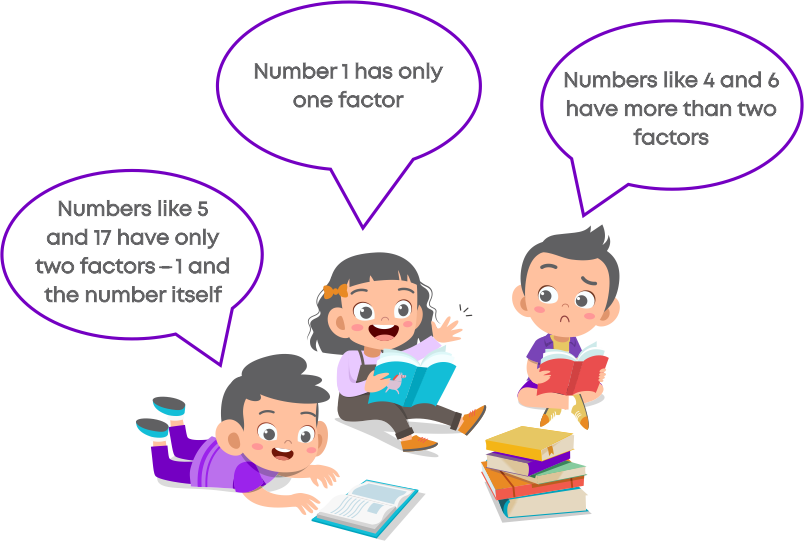The numbers 0 and 1 are neither a prime number nor a composite number.

Some pairs of prime numbers are formed using the same digits.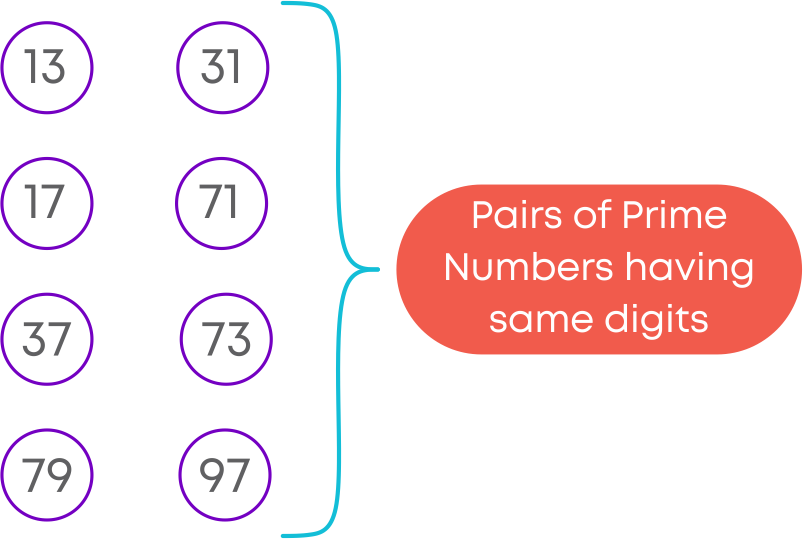Let us learn about the interesting properties of prime numbers:

Two prime numbers whose difference is 2 are called twin primes. Let us find out how many twin primes exist from 1 to 100.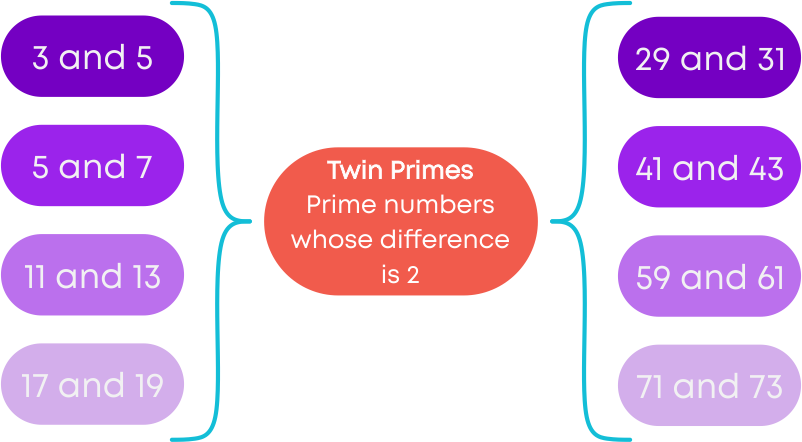Did you know, numbers can be expressed as a sum of two prime numbers? Let us look at some examples.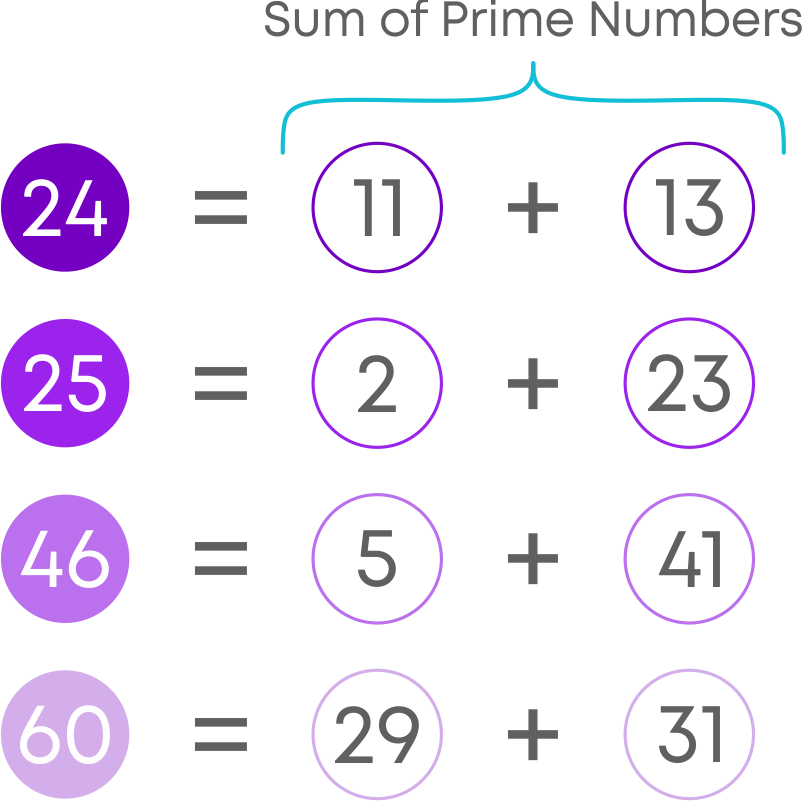When playing with numbers, the first thing you will be taught is to differentiate between even and odd numbers.

The numbers which are multiples of 2 have a special name. They are called even numbers. E.g. 2, 4, 6, 8, …

The numbers which are not multiples of 2 are called odd numbers. E.g. 1, 3, 5, 7, 9..

Is 9876433648 an even number? You need not divide the given number by 2 and check if it is a multiple of 2. Just look at the digit which is at the ones place of the number.

• If the digit at the ones place is 0, 2, 4, 6 or 8, then the number is even.
• If the digit at the ones place is 1, 3, 5, 7, or 9, then the number is odd.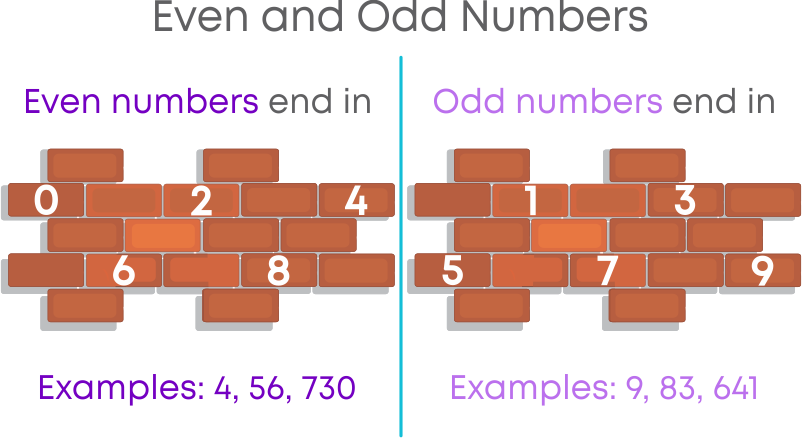Remember, 0 is an even number, 2 is the only number which is both even and prime, and all other prime numbers are odd numbers.

Let us list the properties of addition of even and odd numbers.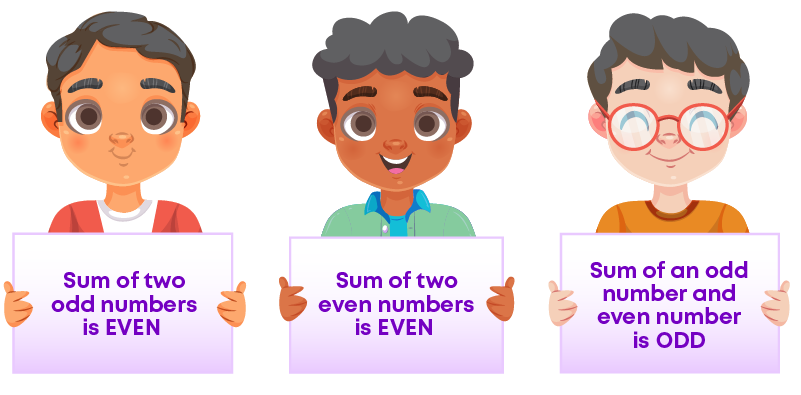For example,

• Sum of two odd numbers 3 and 5 is 8, which is an even number.
• Sum of two even numbers 4 and 6 is 10, which is an even number.
• Sum of an odd number 3 and an even number 6 is 9, which is an odd number.

Let us list the properties of multiplication of even and odd numbers.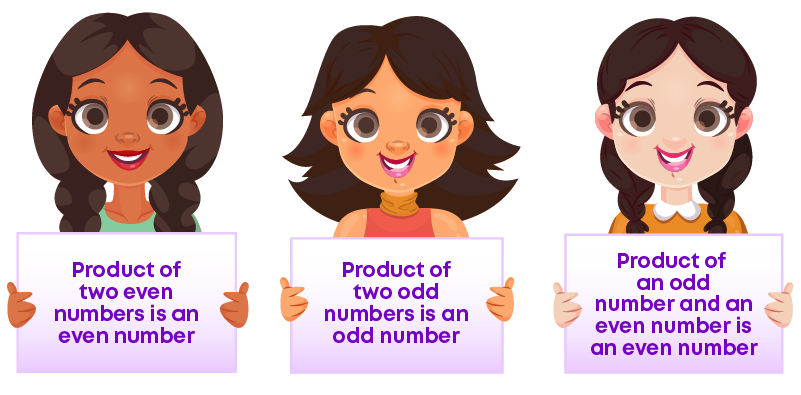For example,

• Product of two odd numbers 3 and 5 is 15, which is an ODD number.
• Product of two even numbers 2 and 4 is 8, which is an EVEN number.
• Product of an odd number 3 and an even number 4 is 12, which is an EVEN number.

#### Divisibility Rules

Divisibility refers to a number's quality of being evenly divided by another number, without a remainder left over.

In other words, a number is said to be divisible by another number if the remainder is 0.

Listed below are the divisibility rules for certain numbers:

Divisibility Rule For 2:

Small numbers can be divided by 2 and checked if they are divisible or not.

But consider a very large number like 98,87,664. Is this number divisible by 2?

EVEN numbers are divisible by 2 and even numbers have the digits 2, 4, 6, 8, or 0 in the one's place, i.e., the numbers are divisible by 2 if the digit at the ones place is 2, 4, 6, 8 or 0.

Divisibility Rule For 3:

1. Add the digits of the number.

2. Check if the sum is divisible by 3.

3. If the sum is divisible by 3, then the number is also divisible by 3. If the sum is not divisible by 3, then the number is also not divisible by 3.

Divisibility Rule For 4:

If the last two digits (i.e., ones and tens) in the number are divisible by 4 then the number is divisible by 4.

Divisibility Rule For 5:

If the digit at the ones place is 0 or 5 then the number is divisible by 5.

Divisibility Rule For 6:

If the number is divisible by both 2 and 3 then it is divisible by 6.

Divisibility Rule For 8:

If the last three digits in the number are divisible by 8 then the number is divisible by 8.

Divisibility Rule For 9:

1. Add the digits of the number
2. Check if the sum is divisible by 9
3. If the sum of digits is divisible by 9, then the number is also divisible by 9. If the sum of digits is not divisible by 9, then the number is also not divisible by 9.

Divisibility Rule For 10:

If the digit at the ones place is 0 then the number is divisible by 10.

Divisibility Rule For 11:

1. Find sum of the digits at odd places (from the right)
2. Find sum of the digits at even places (from the right)
3. Find their difference
4. If the difference is either 0 or divisible by 11, then the number is divisible by 11.

We know the rules for checking the divisibility of given numbers by 2, 3, 4, 5, 6, 8, 9, 10 and 11. Sometimes, the given numbers are divisible by more than one number. For example, all even numbers are divisible by 2. Also, if in the even number, the last two digits are divisible by 4, then it is also divisible by 4.

Let us take the number 2,34,126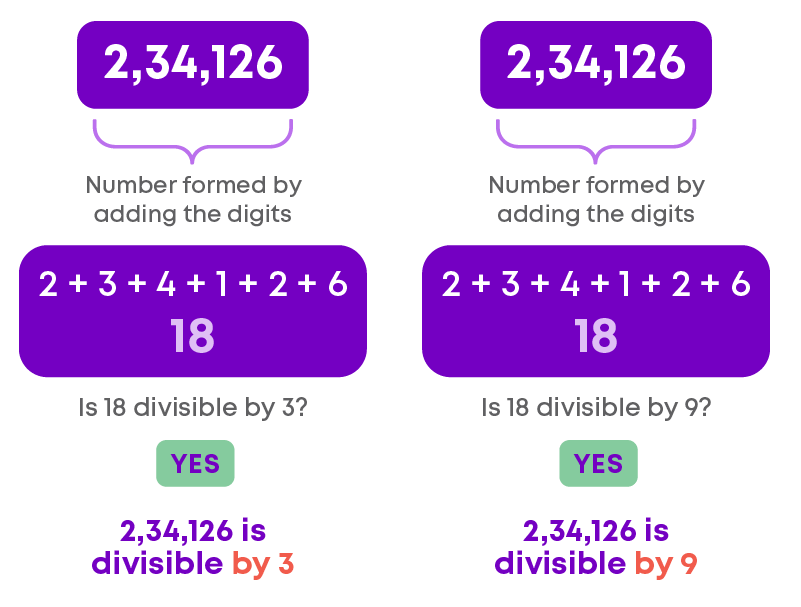Here, the given number is divisible by both 3 and 9.

The divisibility rules are concisely given in the chart below: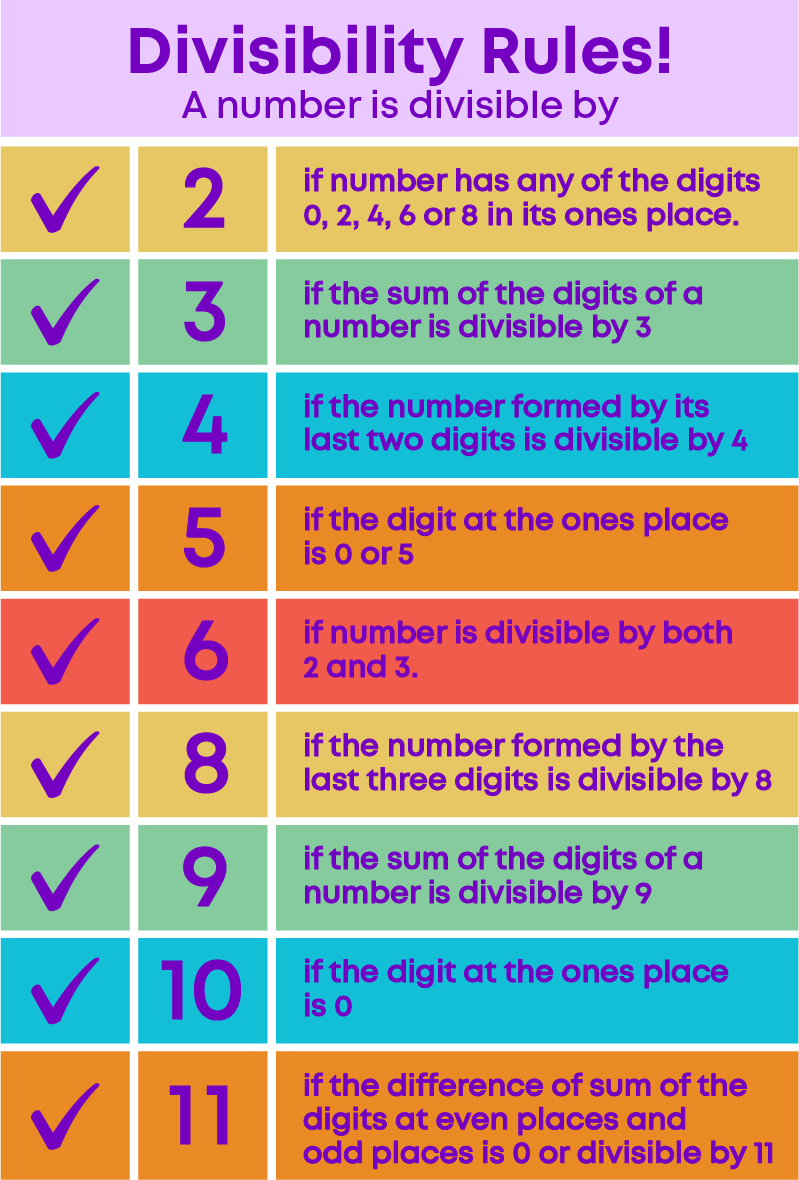Now let us learn how to find the missing digit to make a number divisible by a given number. Follow the steps below:

1. Take unknown digit as 'x'
2. Identify the number which is divisible by the given number
3. Apply the divisibility rule
4. Find the value of 'x'

Consider this example:

35_64 is divisible by 3. How can we find the missing digit?

Given that, the number is divisible by 3. So, we must apply the divisibility rule for 3. As we know, if the sum of digits of the given number is divisible by 3 then the number is divisible by 3.

Sum of the digits = 3 + 5 + x + 6 + 4 = 18 + x

18 + x is divisible by 3.

Multiples of 3 are 3, 6, …., 18, 21, 24, 27, 30…..

If 18 + x = 18, then x = 0.

The number is 35,064

If 18 + x = 21, then x = 3.

The number is 35,364

If 18 + x = 24, then x = 6.

The number is 35,664

If 18 + x = 27, then x = 9.

The number is 35,964

If 18 + x = 30, then x = 12, which is greater than 10 and hence, cannot be a digit of the number.

The possible values x can take is 0, 3, 6 and 9.

What is the smallest digit to replace the blank to make the number divisible by 3? It is 0. By replacing 0 as the value of x, the number becomes 35,064.

Listed below are the properties of divisibility of numbers:

Remember, if a number is divisible by another number, then it is divisible by each of the factors of that number.

If a number is divisible by two numbers, then it is divisible by their product also.

If two given numbers are divisible by a number, then their sum or difference is also divisible by that number.

#### Relationship Between Numbers

A factor of a number is an exact divisor of that number. When we find the factors of two or more numbers, and then find that some factors are the same ("common"), they are the "common factors". 1 is always a common factor of any number (two or more) because 1 is the factor of every number.

Let us see how to find the common factors of 5, 15, and 25.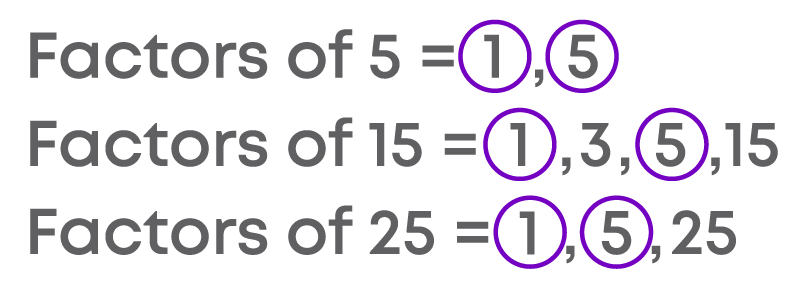For 5, 15, and 25 the factors 1 and 5 are common.

Did you know? Two numbers having only 1 as a common factor are called co-prime numbers.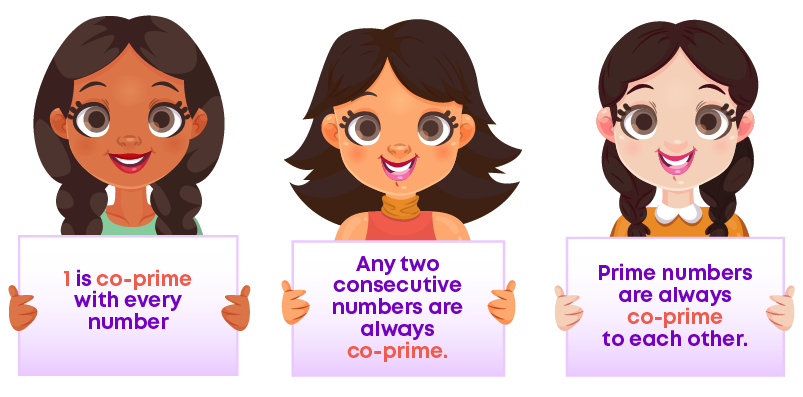A multiple of a number is the product of that number and another number. When we find the multiples of two or more numbers, and then find that some multiples are the same ("common"), then they are the "common multiples".

Let us see how to find the common multiples of 2, 4, and 8.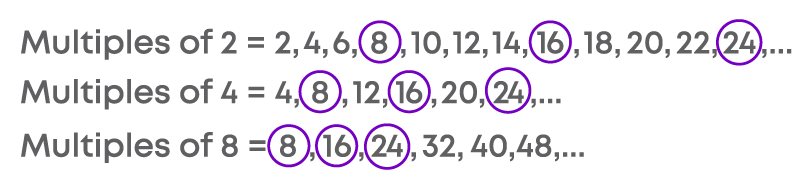Hence, the first three common multiples of 2, 4, and 8 are 8, 16, 24.

#### Prime Factorisation

Do you know the prime factorisation method?

Let us take an example of 12, which can be written as the product of 3 prime numbers:

We can start with different pairs (2, 6) and (3, 4), but we will arrive at the same set of prime factors in the end, which is 2 × 2 × 3. This method of finding the prime factors of a number is called factor tree method.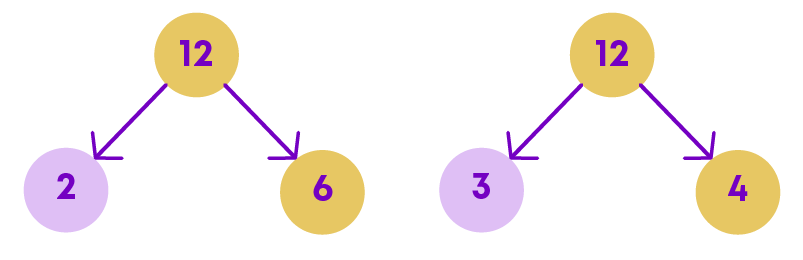The numbers at the end of the factor tree are all the prime factors of the original number. Representing a number as a product of its prime factors is called prime factorisation.

Every number has a unique set of prime factors. No two numbers can have the same prime factorisation.

Now let us consider an incomplete factor tree. Start from the bottom of the tree: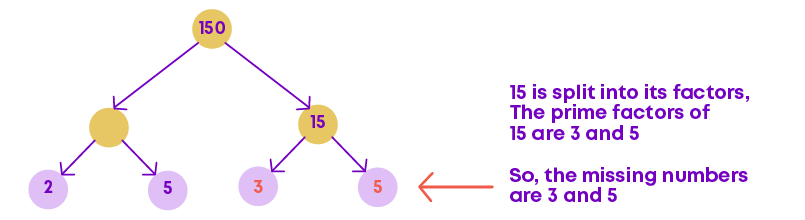Find the next missing number: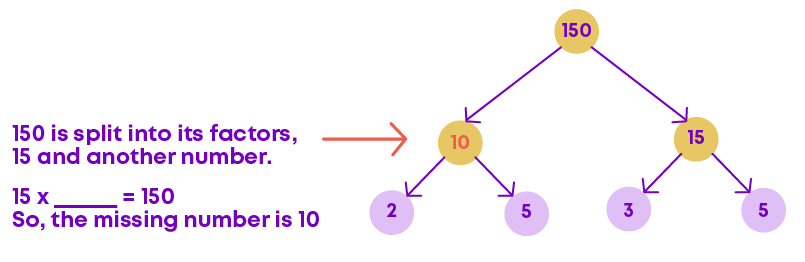This is the complete factor tree.

We saw how to find the prime factorisation of a number by finding the pairs of numbers which when multiplied gives the number. But for larger numbers, it may not be easy to find such pairs of numbers. To prime factorise such numbers easily, we use division method.

The division method of prime factorisation involves the following steps:

• Start dividing the number by the least prime factor
• Continue the division until we get 1 as the result
• Collect all prime numbers and write them as a product

Consider prime factorising 48 using the division method: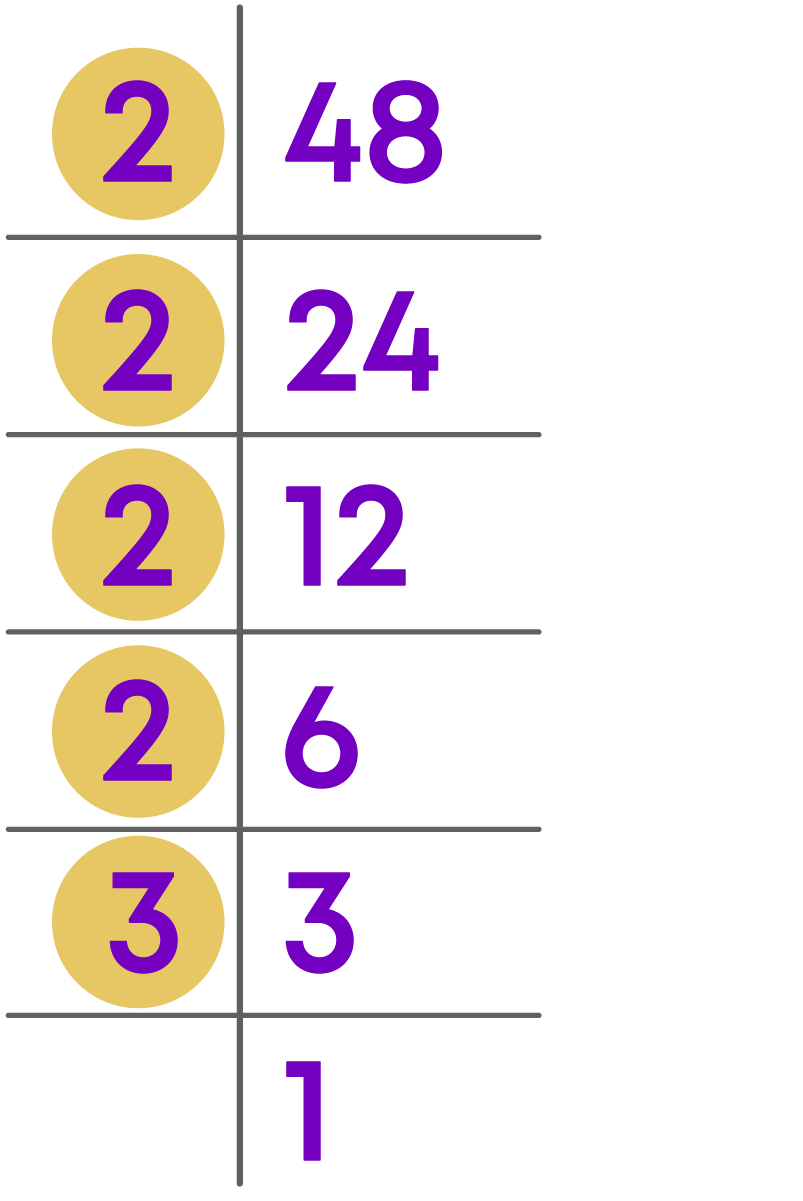Prime factorisation of 48 = 2 × 2 × 2 × 2 × 3

#### LCM and HCF

The Highest Common Factor (HCF) of two or more given numbers is the highest (or greatest) of their common factors. It is also known as Greatest Common Divisor (GCD).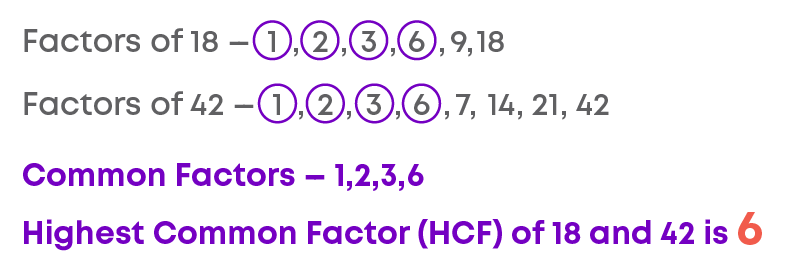Remember these points:

• HCF of co-prime numbers is 1
• HCF of two consecutive numbers is 1
• HCF of two consecutive even numbers is 2
• HCF of two consecutive odd numbers is 1
• HCF of two or more numbers cannot be greater than any of the numbers

Let us learn how to find the HCF of a number using prime factorisation.

Prime factorisation of 18, 54 and 81 is as follows:

1. Prime factorise each number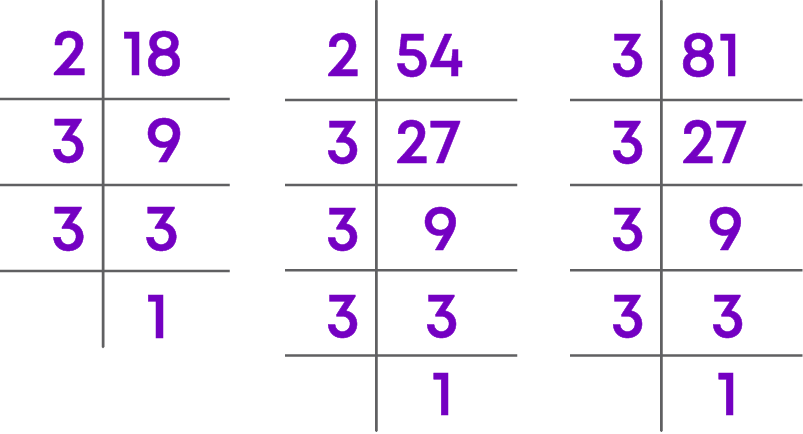2. Identify common prime factors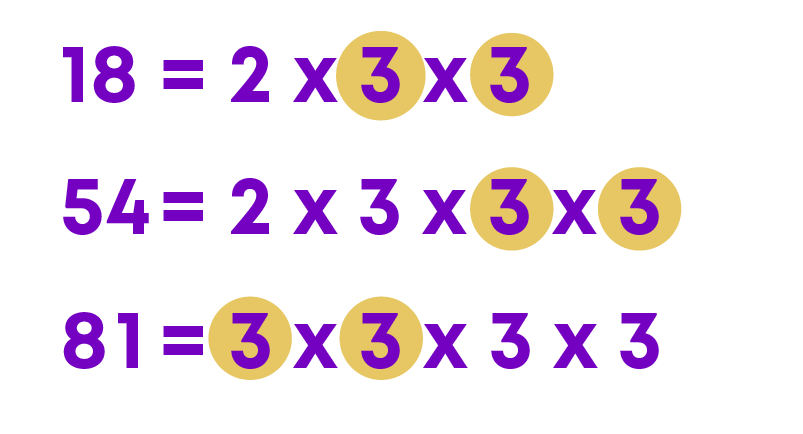3. The product of common prime factors gives the HCF

HCF = 3 × 3 = 9

Hence, HCF of 18, 54 and 81 is 9.

We use HCF to solve different types of problems. Based on the type of the problem, the strategy to solve it will differ.

• To find the greatest number that divides exactly

Strategy

Find the HCF of the numbers.

To find the greatest number that leaves the same remainder

Strategy

1. Subtract the remainder from the numbers

2. Find the HCF of new numbers

To find the greatest number that leaves a different remainder

Strategy

1. Subtract the respective remainder from each number

2. Find the HCF of new numbers

The Lowest Common Multiple (LCM) of two or more given numbers is the lowest (or smallest or least) of their common multiples.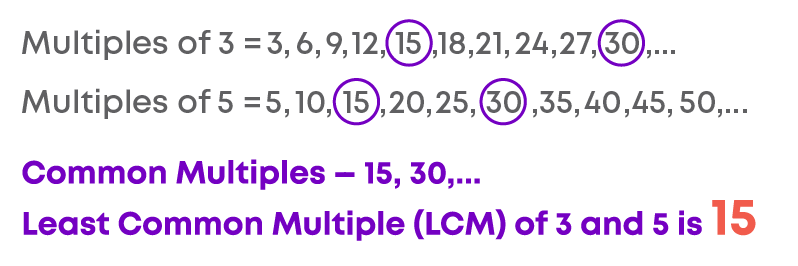Remember these points:

• LCM of two or more numbers cannot be less than any of the numbers
• LCM of co-prime numbers is the product of the numbers
• When we have two numbers, a number, and its factor, then their LCM will be the number itself

You know how to find the LCM of two numbers by listing their multiples and identifying the least common multiple. This may be a time-consuming process for large numbers. In such situations, we use prime factorisation method.

Let us find the LCM of 18, 24 and 36.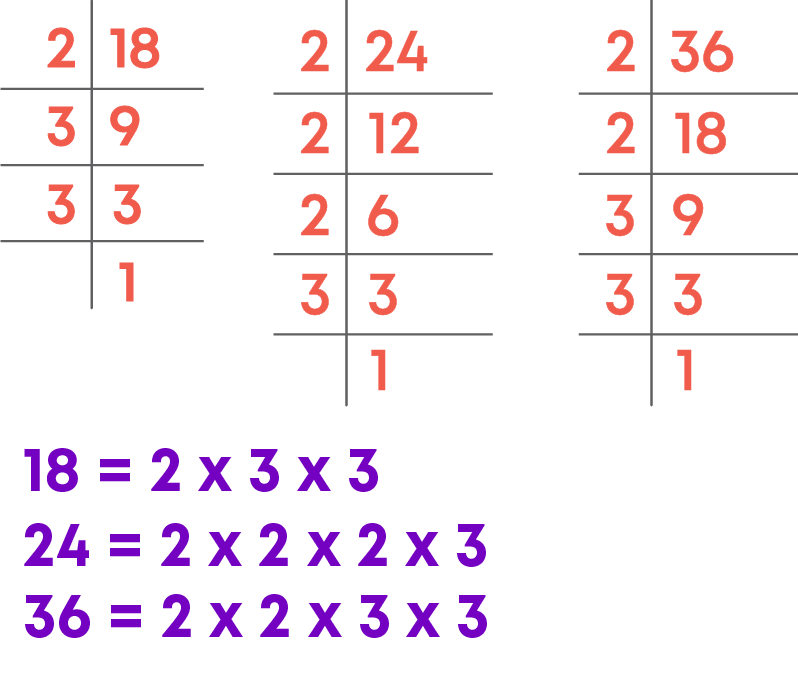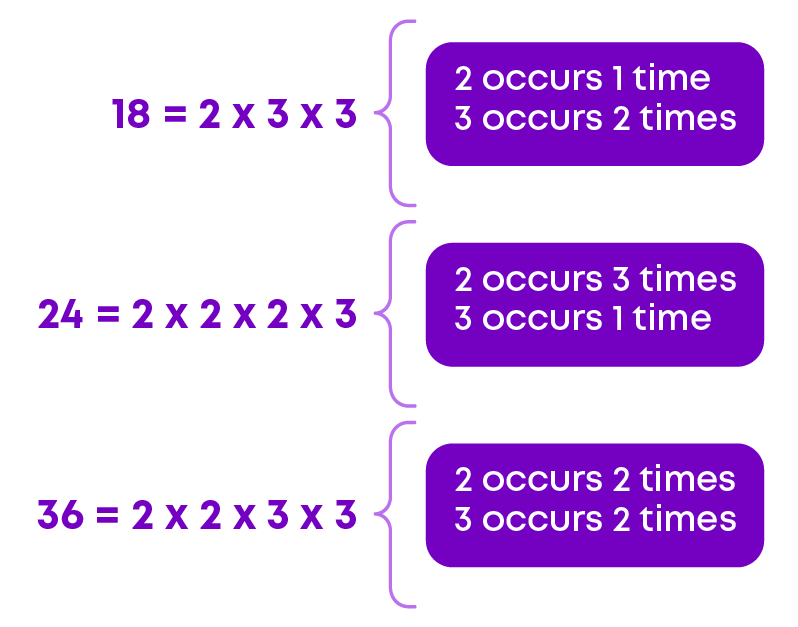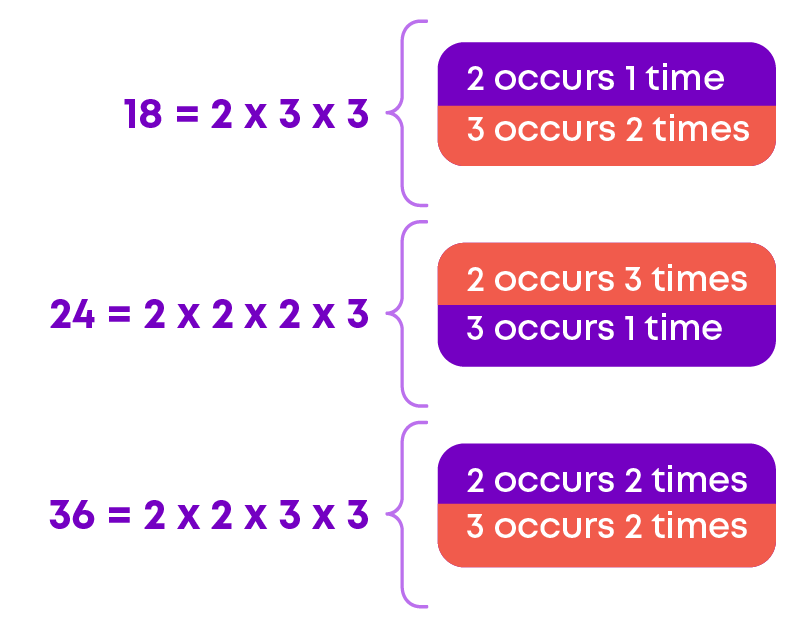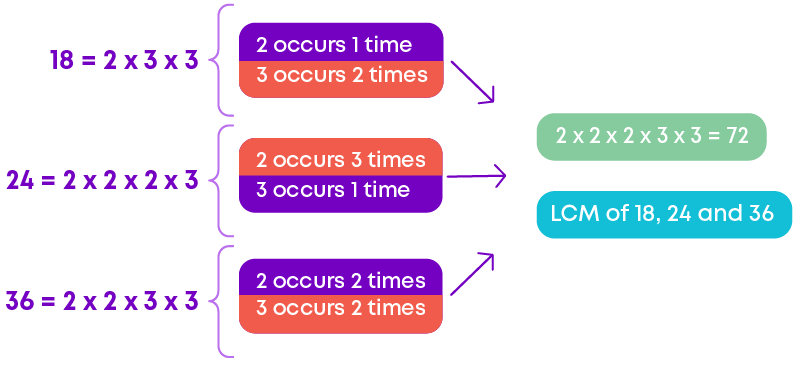Thus, LCM of 18, 24 and 36

= 2 × 2 × 2 × 3 × 3

= 72

LCM can also be found using the division method. Consider the numbers 18, 25 and 30.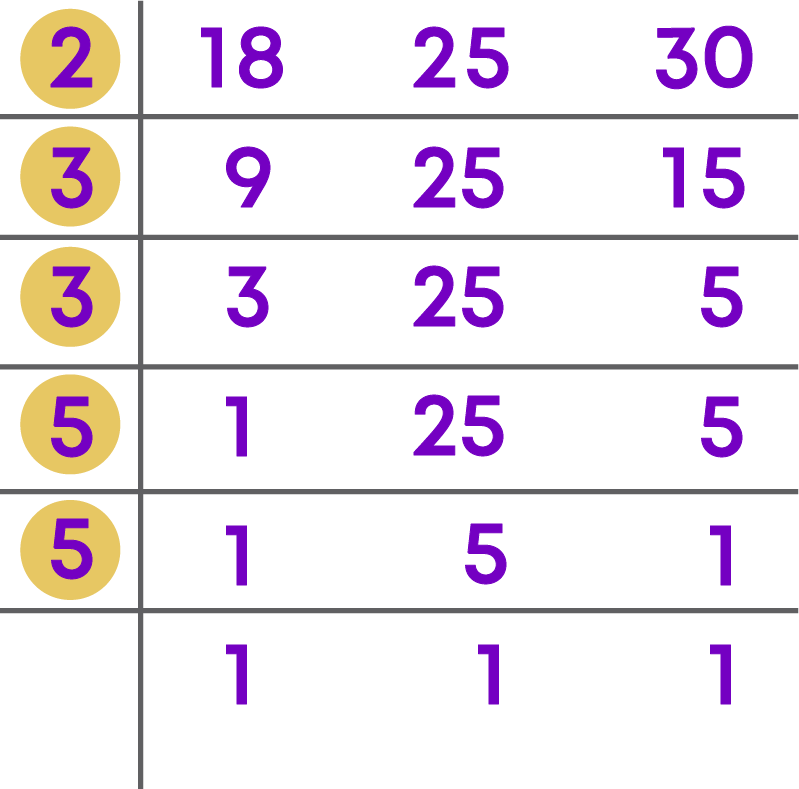LCM is the product of all these prime numbers.

LCM of 18, 25 and 30 = 2 × 3 × 3 × 5 × 5 = 450

We use LCM to solve different types of problems.

• To find the smallest number which is exactly divisible by the given numbers
• To find the smallest number, upon decreasing by a quantity, is exactly divisible by the given numbers
• To find the smallest number, upon increasing by a quantity, is exactly divisible by the given numbers

Now that we know HCF and LCM, let us look at the relationship between them. Consider the numbers 48 and 60.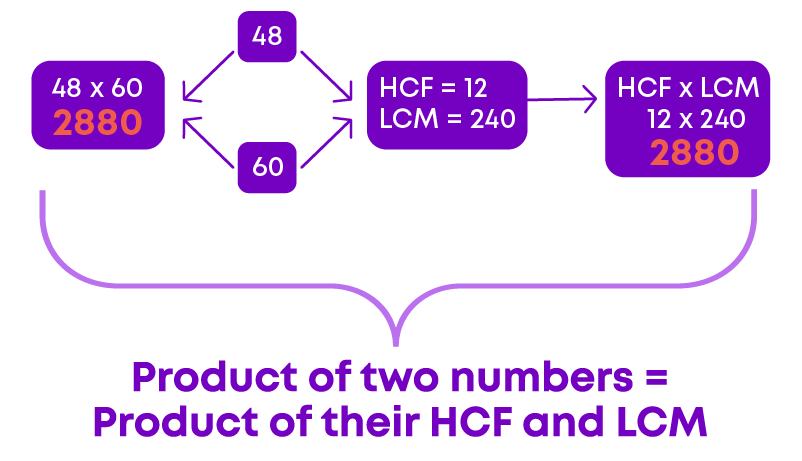LCM of two numbers = product of two numbers / HCF of two numbers

HCF of two numbers = product of two numbers / LCM of two numbers

#### Common Errors

The following are the topics in which students make common mistakes when dealing with numbers:

• 1. Do not omit 1 and the given number in the list of factors
• 2. Confusion between odd and prime numbers
• 3. Prime factor and factor
• 4. HCF is never ‘0’
• 5. HCF is the product of common factors
• 6. LCM by prime factorisation method

#### Do Not Omit 1 And The Given Number In The List Of Factors

What are the factors of 6? Are they 2 and 3? No! 1 and the number itself are also factors of the given number. So, the factors of 6 are not 2 and 3 alone.

The factors of 6 are 1, 2, 3 and 6.

#### Confusion Between Odd And Prime Numbers

Which of the following are prime numbers?

2, 3, 4, 5, 7, 9, 13, 16.

The prime numbers are 2, 3, 5, 7 and 13. Many times, the terms ‘prime’ and ‘odd’ numbers may cause confusion. We know that 2 is the only even prime number and all other prime numbers are odd. That does not mean that all the odd numbers are prime numbers.

9 is not a prime number since it has more than 2 factors, 1, 3 and 9. For better understanding let us categorise the numbers as follows.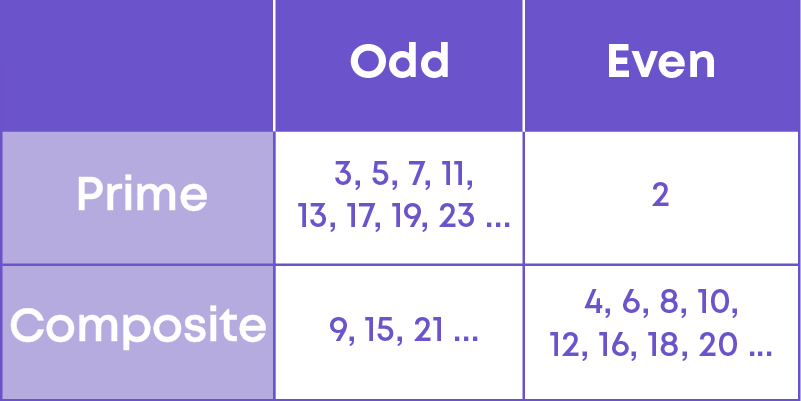#### Prime Factor And Factor

20 is divisible by 4. Can you say that 4 is a prime factor of 20? No! 4 is a factor, but it is not a prime number. The prime factors of 20 are 2 and 5.

20 = 2 × 2 × 5

Remember! Prime factor is the factor of a number that is itself, a prime number.

#### HCF Is Never ‘0’

4 = 2 × 2

9 = 3 × 3

What is the HCF of 4 and 9?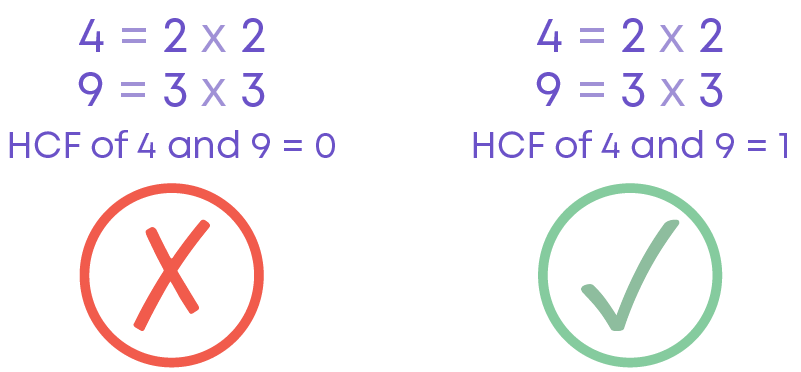4 and 9 do not have any common factors. Can you say that the HCF is ‘0’? No!

‘0’ cannot be a factor of any number, hence, it cannot be the HCF of any two numbers.

Also, you know that 1 is a factor of every number. We can write 4 and 9 as:

4 = 1 × 2 × 2

9 = 1 × 3 × 3

Hence, 1 is the common factor of 4 and 9.

HCF (4, 9) = 1.

#### HCF Is The Product Of Common Factors

Care should be taken while finding the HCF. Consider the numbers 4 and 12.

4 = 2 × 2

12 = 2 × 2 × 3

What is HCF of 4 and 12? Is it 2? No!

HCF is not the common factor, but it is the product of all the common factors. We can see that 2 is repeated twice.

Hence, HCF of 4 and 12 = 2 × 2 = 4.

#### LCM By Prime Factorisation Method

Care should be taken while finding LCM by the prime factorisation method. Consider the numbers 70 and 84.

70 = 2 × 5 × 7

84 = 2 × 2 × 3 × 7

What is the LCM of 70 and 84?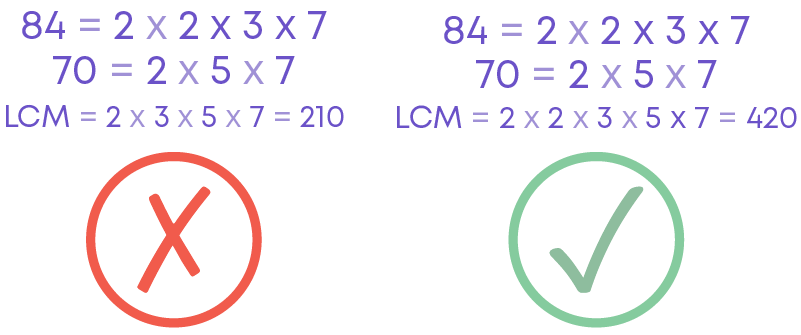The LCM of two numbers is the product of the prime factors that occurs the maximum number of times in any of the numbers.

2 occurs a maximum number of 2 times in the prime factorisation of 84.

Hence, LCM (70, 84) = 2 × 2 × 3 × 5 × 7 = 420.

#### Conclusion

Now you know about numbers, their properties, divisibility, and the relationship between numbers. Can you solve the puzzle below?

Replace the question marks with the correct answer.